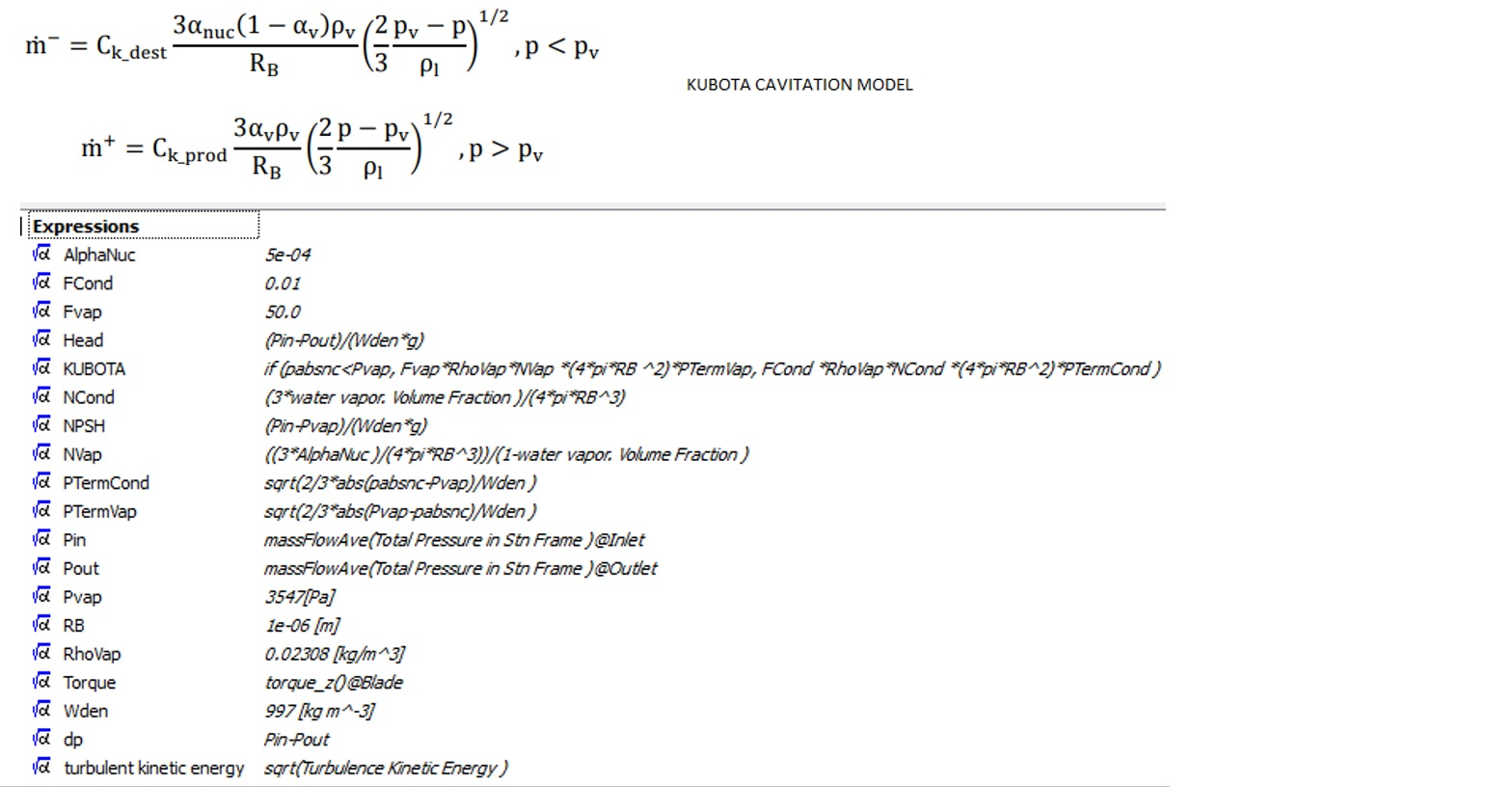## Fluids

•mckoz
Subscriber

Hi all,

I am attempting to create a conditional statement in CFX-Pre using CEL, either the step() function or an if() statement. Basically, I am running a Non-Newtonian simulation and some of the results of my simulation fall outside of the shear rate range specified within the CFX Non-Newtonian model. I am attempting to assign a constant value to the Dynamic Viscosity if the shear strain rate (sstrnr) is less than 0.001 s^-1, which is the lower limit of CFX. If the shear strain rate is greater than or equal to 0.001 s^-1, I would like for my Non-Newtonian model to be applied. I would like to do this because there are a few areas in my geometry that have shear strain rates lower than 0.001s^-1, and it is yielding unrealistically high viscosity results. Thus, for these regions, I would just like to assign some constant value of viscosity to make up for this.

Does anyone have any ideas about how I could do this? Thanks in advance.

•raul.raghav
Subscriber

I assume you are trying to model the Non-Newtonian fluid using the Ostwald de Waele model. I could be wrong but I don't think you can assign a specific value for dynamic viscosity if the sstrnr goes below a certain value.

If I were in your shoes, I would write an expression for the the dynamic viscosity and bound the lower limit of the shear strain rate such that if sstrnr < 0.001 s^-1, I'd assume it to be 0.001 s^-1. So wherever your shear strain rate would be less than 0.001 s^-1, the dynamic viscosity will be calculated at sstrnr = 0.001 s^-1. The following CEL expressions would bound the lower and upper limits of sstrnr and implement the dynamic viscosity as an expression.

dynVisc = K * ((L * bSSTRNR)^(n-1))

bSSTRNR = if(sstrnr < 0.001 [s^-1], 0.001 [s^-1], cond)

cond = if(sstrnr > 1000 [s^-1], 1000 s^-1, sstrnr)

K - viscosity consistency; L - time constant; n - power law index; bSSTRNR - bounded sstrnr

•nehir
Subscriber

Hello Dear Raul

I try to modelling KUBOTA cavitation model in CFX. But the Cfx cannot solve problem. I will send you me expression and model file.

Could you help me?•DrAmine
Ansys Employee

Do you have problems with the expressions or with the setup itself?